TUTORIALS
TUTORIALS HOME

GENERAL MATH
NUMBER SETS
ABSOLUTE VALUE & INEQUALITIES
SETS & INTERVALS
FRACTIONS
POLYNOMIALS
LINEAR EQUATIONS
GEOMETRY
FINITE SERIES
TRIGONOMETRY
EXPONENTS
LOGARITHMS
INDUCTION

CALCULUS
LIMITS
DERIVATIVES
RELATED RATES & OPTIMIZATION
CURVE SKETCHING
INTEGRALS
AREA & VOLUME
INVERSE FUNCTIONS

MAIN
HOME
TESTS
TUTORIALS
SAMPLE PROBLEMS
COMMON MISTAKES
STUDY TIPS
GLOSSARY
CALCULUS APPLICATIONS
MATH HUMOUR

### EXPONENTS TUTORIAL

Exponential expressions have the form xa, where x is the base and a is the exponent. xa refers to the product,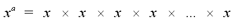where there are 'a' factors of the term x. For example,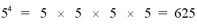The exponential expression x0 is equal to 1 for all x that are not equal to 0. When 0 is raised to an exponent of 0 (00), the expression is undefined.

Exponents can take on different values, such as negative integers and fractions. The following formulas show how to calculate these types of exponential expressions.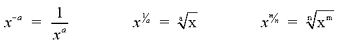Note: In general,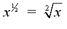is simply written as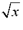. In the expression x1/a, if a is even, then x cannot be negative or it will be undefined.

# Examples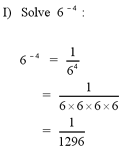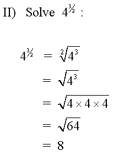# Laws of Exponents

The following laws must be followed when multiplying and dividing powers. Laws a) and b) only apply when the two powers have the same base. Laws c) and d) only apply when the two bases are raised to the same exponent.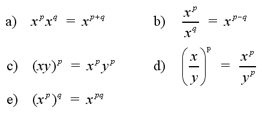# Examples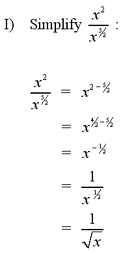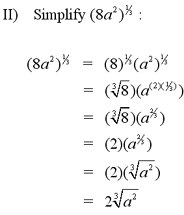Note: For a complex example of simplifying an exponential expression, see question #1 in the Additional Examples section below.

1 | Simplify the exponential expression

| Top of Page |

COURSE HOMEPAGES
MATH 1036
MATH 1037

FACULTY HOMEPAGES
Alex Karassev
Ted Chase